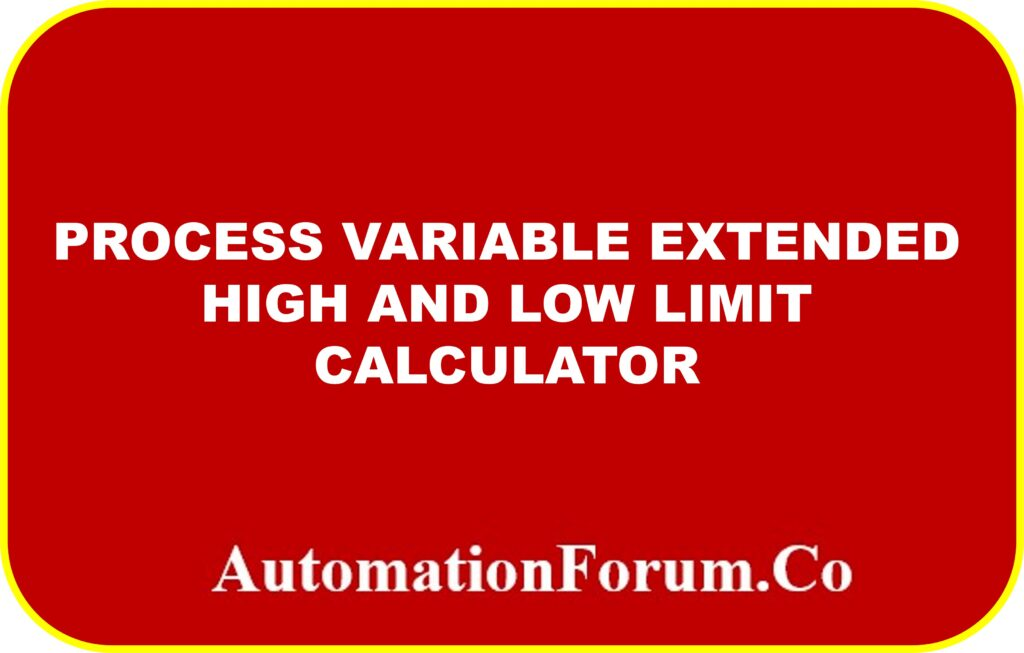# EXtended high and low limit calculator## What is Lower Range Value (LRV) of a transmitter?

Lower range value is the smallest measurement that a device can calibrated to measure. Another name for the LRV is Zero.

## What is Upper Range Value (URV) of a transmitter?

Upper range value is the maximum measurement that a device can be calibrated to measure. Another name for the URV is Span.

## What is extended lower limit value of a transmitter?

In the event that the process variable falls below the instrument calibration range, the extended low range is employed to measure the process variable’s lower value.

## What is extended higher limit value of a transmitter?

In the event that the process variable rises above the instrument calibration range, the extended high range is employed to measure the higher value of the process variable.

## Formula for calculating EHL and ELL value

ELL value = LRV-((URV-LRV)*ELL LIMIT %)

EHL value = ((URV-LRV)* EHL LIMIT %)+URV

## Example calculation:

For instance, the level of a tank with a range of -200 to 800 inch  is the process variable to be monitored with Extended Low Limit (ELL) is set to 5% Extended High Limit (EHL) is set at 3%.

## ELL value calculation

The Range is then 100 and the LRV is -200 inch

URV is 800 inch

ELL value = LRV-((URV-LRV)*URV LIMIT%)

ELL value =-200-((800-(-200))*(5/100))

ELL value =-200-(1000*0.05)

ELL value = – 250 inch

The Extended Low limit value can be -250 inch if the Extended Low Limit (ELL) is set to 5%.

## EHL value calculation

EHL value = ((URV-LRV)* EHL LIMIT%)+URV

EHL value = ((800-(-200))*(3/100))+800

EHL value =  (1000*0.03)+800

EHL value = 830 inch

The Extended high limit value  can reach 830 inch if the Extended High Limit (EHL) is set at 3%.

## Extended high and low limit calculator

The below calculator used to calculate the Extended high and low limit of the process variable